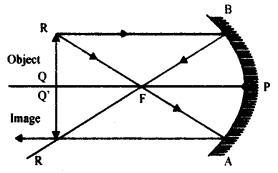# A spherical mirror produces an image of magnification-Ion a screen placed at a distance of 40 cm from the mirror:

A spherical mirror produces an image of magnification-Ion a screen placed at a distance of 40 cm from the mirror:
(i) Write the type of mirror.
(ii) What is the nature of the image formed?
(iii) How far is the object located from the mirror?
(iv) Draw the ray diagram to show the image formation in this case.

(i) The mirror is concave.
(ii) The image formed is real and inverted and of the same size as that of the object.
(iii) In this case, the object is placed at the centre of curvature of the mirror, so the object distance is equal to the image distance. Here, the screen is placed 40 cm from the mirror.
Image distance = Object distance = 40 cm.
(iv)